Copyright (c) 2019
All Rights Reserved

amortization.com Ltd.
Burlington, Ontario
CANADA

905-639-0374
905-407-7988

info@amortization.comAmortization Pro for iPhone/iPad/iPod

 Understanding an Amortization Schedule

An amortization schedule is a record of your loan or mortgage payments, showing, payment number, payment date, payment amount (and a breakdown of how much is principal and how much is interest) and the balance owing after that payment has been made. For a detailed explanation continue reading below. For a different perspective of an amortization schedule, click here.

Assume you borrow \$10,000 from a lender for an automobile purchase. The lender offers you an Annual Interest Rate (AIR) of 12% and you agree to pay \$350 each month. A 12% Annual Interest Rate was chosen purposely, to demonstrate the simplicity of an amortization schedule. Twelve percent per year is one percent per month. The lender advances you or gives you the \$10,000 on June 15th (June 15th is the advance date) and on July 15th (one month later) the first monthly payment is due.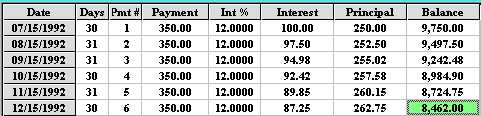At the end of the month, the lender multiplies the monthly interest factor times the outstanding balance and the interest owing for the first month is \$100.00 (.12 x 10,000/12). Only \$250 of the monthly payment is applied towards the principal and the balance owing to the Lender immediately after you give them the \$350 payment is \$9,750.00. When any payment is given to a lender the interest for the use of the money is calculated and taken first, the remaining amount goes towards reducing the principal. If the payment is not large enough to cover the interest, a negative amortization schedule is produced and the principal owing starts to increase. The interest shortfall is added to the balance.

Next months payment is due on August 15th, the balance owing is \$9,750 and the interest owing for the use of the money for the second month is 0.01 x 9,750 = 97.50 thus \$252.50 is applied against the loan or mortgage. The balance owing immediately after that second payment is \$9,497.50. This is how an amortization schedule is calculated. If you want to see how it looks printed, (Click Here). If you have the time, the first six months of the schedule could be manually calculated as shown using the MORTGAGE2 PRO software.

(Click Here) for a summary of the types of amortization schedules the MORTGAGE2 PRO calculates.

The software automatically calculated the amortization period as 2.82 years because the payment was specified..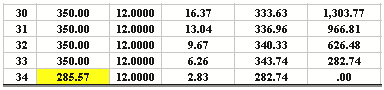The 2.82 years is 33 payments of \$350 plus a 34th payment of \$285.57 The 34th and last payment of 285.57 is because of the mathematics. You cannot always specify an exact payment to the end of the amortization period. The last payment sometimes is less than the prior payments in order to have the equations balance.

(See Schedule)

After the sixth payment, on December 15th 1992 the balance owing is \$8,462.00. You are made aware that \$87.25 was the interest cost for the period Nov 15 to Dec 15 and the balance owing is decreased by \$262.75. If on December 15th 1992 you made the 6th payment in the amount of \$350 + \$8,462 that would be called the balloon payment and the loan would be finished.

This method is used for most loans and mortgages except for Lenders, that use the exact day monthly schedule. The only difference between a loan and a mortgage in Canada, is how the interest factor is calculated. All mathematical equations for loans and mortgages are identical. The only difference is the numerical value of the interest factor. In the USA, most mortages and loans are monthly payments and monthly compounding, thus the interest factors are identical. A mortgage is just a specific name for a loan used to purchase a house. In this example, the interest factor (0.12/12 = 0.01) or monthly interest of 0.01 was purposely selected in order to easily show the monthly interest calculation. In Financial circles the phrase, monthly interest factor and monthly interest are often used to mean the same thing.

To be concise, the above example is a fixed rate monthly payment mortgage with monthly compounding and blended payments. This is the most common method used for American mortgages and both Canadian and American consumer loans such as automobile loans. Canadian mortgages use semi-annual compounding, however the explanation is identical. The payment is the same every month but more and more of the payment is applied towards the principle repayment as time progresses. This is why it is called blended.

Interest Rate Jargon

The Annual Interest Rate (AIR) is sometimes called the Stated Annual Interest Rate therefore don't be alarmed by different names. In Financial circles it is customary to quote Annual Interest Rates and designate the conversion or compounding frequency. If the Compounding frequency is mentioned along with the AIR then the AIR is also referred to as the Nominal Interest Rate or Nominal Annual Interest Rate.

The words, conversion and compounding are often used as synonyms (means the same thing). Don't be misled. Compounding and calculation are not the same thing! Do not fall in the trap of thinking that compounding means calculation. When a loan or mortgage payment is due at the end of a period of time, an interest calculation is performed using an interest factor. If the frequency of the payments are monthly then an interest calculation is done every month. This last statement is worth repeating. In other words, if the frequency of payments are weekly then an interest calculation (using a weekly interest factor) is done every week. Each time a payment is due, an interest calculation is performed prior to the payment. The numerical value of the interest factor is determined by the compounding frequency and the number of days in the year and the particular formula used.

For a given loan or mortgage with a fixed interest rate the interest factor does not change from one payment to the next, unless it is an exact day monthly payment mortgage (choose a 365 day year in the software package) or an adjustable rate mortgage (ARM). Once you calculate the first line of an amortization schedule you can calculate the rest of the schedule the same way. Think of the words, compounding or conversion as adjectives that help describe the interest factor. In other words, conversion or compounding are interchangeable words used to describe how the interest factor was calculated.

In the above example the 12% Annual Interest Rate with monthly compounding could also be referred to as a 12% nominal interest rate because the compounding frequency was specified. The monthly interest factor in the example above was arrived at by dividing the Annual Interest Rate by 12, the number of payments per year.

If the compounding was semi-annual (Federal Canadian law for a Canadian mortgage), the monthly interest factor in the above example would be 0.009758794179192 (which was not arrived at by dividing by 12). You do not need to understand the mathematical formula used to calculate this interest factor, 0.009758794179192 in order to use it in a monthly calculation, just as you do not need to be an auto mechanic to drive an automobile.

To understand the phrase, effective interest rate (EIR), think of semi-annual compounding as a vestige of the lending practices in effect (100 years ago) in the early farming communities. Then Farmers were the main borrowers from the Banks. Farmers initially borrowed money for crops on a six month basis. Thus 12% per year would be six percent half yearly or semi-annually. A six percent yield after six months is shown mathematically as 1.06. Thus 1.06 x 1.06 = 1.1236 thus a 12.36% effective interest rate (an annual basis is always assumed when speaking of effective interest rates). It is interesting to note that 1.0097587 multiplied by itself twelve times is exactly equal to 1.1236 which is all that semi-annual compounding is meant to be, no more no less. In other words the .0097587 factor used each month is effectively the same as if a semi-annual factor of 0.06 was used in the calculation of the Effective Interest Rate.

Another way of stating it is as follows; 1.06 multiplied by itself twice is mathematically the same as 1.0097587 multiplied by itself twelve times. Semi-annual compounding is a mathematical technique that makes it appear as if interest calculations and payments are done semi-annually even though in reality the interest calculations and payments are being done monthly, biweekly or weekly. The technique ensures that the effective interest rates are identical thus the amount of interest paid is identical.

Think of the effective interest rate (EIR) as a way of allowing you to compare apples and oranges. A lender gives you a \$10,000 loan at an interest rate of 11.7106% with monthly compounding, amortized for one year. The monthly payment would be \$887.13 and after 12 payments you would have paid back the loan plus \$645.62 in interest. Now suppose a different Lender offered you an interest rate of 12% but with semi-annual compounding and allowed you to pay back the same \$887.13 per month. Would you be able to verify that after 12 payments you would have paid back the entire loan plus \$645.62 in interest?. The two Lenders are offering you the EXACT conditions because the Effective Interest Rates (EIR) are identical at 12.36%. If Lenders quoted effective interest rates, you would not have to be concerned with compounding jargon.

The two loans are identical because the EIRs are the same. In other words 11.7106% with monthly compounding is exactly the same as 12% with semi-annual compounding. The two amortization schedules (11.7106% vs 12.0000%) are identical. If the header information at the top of the page was missing, you would not be able to tell the 12.0000% schedule from the 11.7106% schedule.

There is a more compelling reason for understanding amortization schedules and effective interest rates. Effective interest rates are always on an annual basis thus it is very easy to compare mortgages and other investment vehicles such as bonds or mutual funds because the latter two financial vehicles are usually compared on an annual basis (annual yields).

If you lent out \$10,000 at an Annual Interest Rate of 12% with semi-annual compounding for a period of one year in effect you would be receiving an annual rate of return of 12.36%. From (Screenshot 1) you may be alarmed to see that the total interest after 12 payments is only \$645.60

If you take the monthly payment and stuff it under the mattress each month, then \$645.60 is all that your investment will yield. However if you reinvest that monthly payment each month at 12% with semi-annual compounding then the negative amortization schedule in (Screenshot 2) shows that the \$10,000 will accumulate to \$11,236 (11,235.98 shown) in twelve months for an annual yield of 12.36%. A negative amortization schedule (easily performed by MORTGAGE2 PRO) is one way of performing a present value/future value calculation by setting all the payments equal to zero . From (Screenshot 3) (calculated from the MORTGAGE2 PRO Utility Menu) you can see a Present Value/Future Value calculation also gives the same yield or annual return of 12.36% (I=12.360000).

It is important to understand the EIR concept because that is the true yield to be used when comparing financial vehicles. If you know the annual interest rate and the compounding frequency then you must be able to convert it to an EIR. From (Screenshot 4) it can be seen that the more frequent the compounding the larger the monthly interest factor thus a larger yield (EIR) for the Lender. From (Screenshot 4) the lender can achieve a yield from 12% to 12.75% by choosing the appropriate compounding frequency to give a larger EIR. Thus an Annual interest Rate alone is not sufficient to accurately spell out the Yield. The Yield is often used to refer to the effective interest rate (EIR) .

Summary Of Interest Rate Terminology

A lender quoting a rate as, "Annual Interest Rate of 12%", is misleading you. You require more information. The four rates below (in blue) are identical, because the effective interest rate for all of them is 12.36%. This means, at the end of one year, of making the exact same monthly payments, you pay \$645.60 in interest regardless of which of the four rates chosen below.

Nominal Annual Interest Rate of 12%, compounded semi-annually

Nominal Interest Rate of 12%, compounded semi-annually

Nominal Annual Interest Rate of 11.7106%, compounded monthly

Nominal Interest Rate of 11.7106%, compounded monthly

It is simpler to quote a loan with an effective interest rate of 12.36% rather than trying to read through all the confusing wordage?

Do not confuse the effective interest rate, EIR, with the APR or TCOB. It has been said that "Knowledge is power."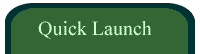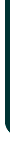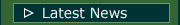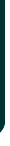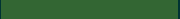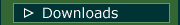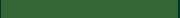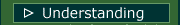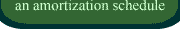VIDEOSamortizationdotcom Mortgage Calculator for iPhone

Introduction to Canadian and American Mortgages

Seminar on prepaying principal (Part A)

Seminar on prepaying principal (Part B)

Global TV Interview regarding 40 Year MortgagesLook for this logo on the Apple Store!

 < Go Back